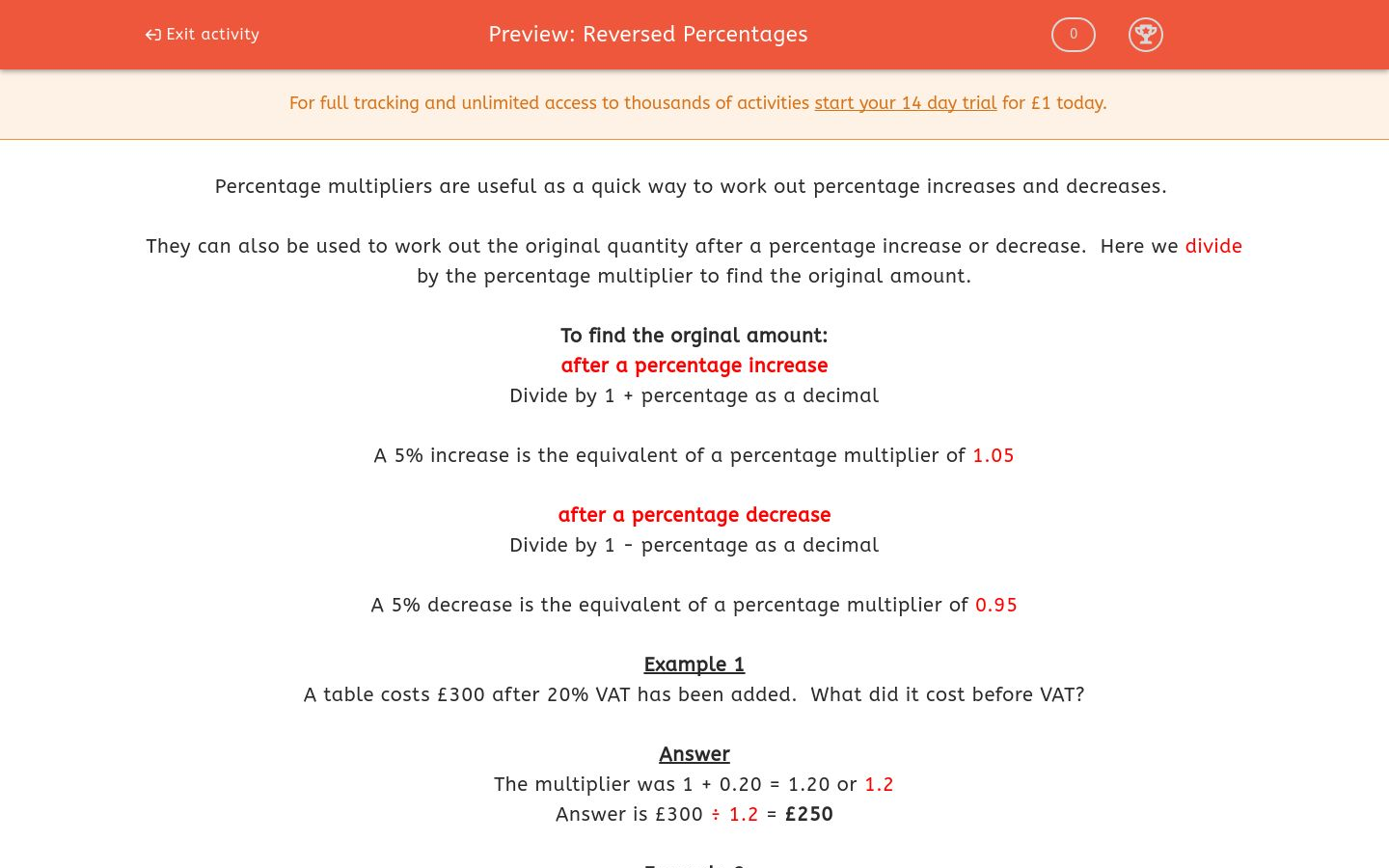# Reversed Percentages

In this worksheet, students use percentage multipliers to work out the original price after a percentage increase or decrease.Key stage:  KS 4

Curriculum topic:  Ratio, Proportion and Rates of Change

Curriculum subtopic:  Set Up, Solve and Interpret Answers in Growth and Decay Problems

Difficulty level:### QUESTION 1 of 10

Percentage multipliers are useful as a quick way to work out percentage increases and decreases.

They can also be used to work out the original quantity after a percentage increase or decrease.  Here we divide by the percentage multiplier to find the original amount.

To find the orginal amount:

after a percentage increase

Divide by 1 + percentage as a decimal

A 5% increase is the equivalent of a percentage multiplier of 1.05

after a percentage decrease

Divide by 1 - percentage as a decimal

A 5% decrease is the equivalent of a percentage multiplier of 0.95

Example 1

A table costs £300 after 20% VAT has been added.  What did it cost before VAT?

The multiplier was 1 + 0.20 = 1.20 or 1.2

Answer is £300 ÷ 1.2 = £250

Example 2

A tennis racket costs £76.50 in a 15% sale.  What did it cost before the discount was applied?

The multiplier was 1 - 0.15 = 0.85

Answer is £76.50 ÷ 0.85 = £90

Use a percentage multiplier to calculate the original price.

A child's car seat costs £68.25 after 5% VAT has been added.  What was its price before VAT?

£65

£68.25

£61.75

£65.50

Use a percentage multiplier to calculate the original price.

A car costs £11400 after 20% VAT has been added.  What was the price before VAT?

£1900

£9520

£9500

£11400

Use a percentage multiplier to calculate the original price.

A football costs £10.80 in a 10% sale.  What did it cost before the sale?

£12

£10.90

£10.80

£12.20

Use a percentage multiplier to calculate the original price.

A suitcase costs £36 in a 25% sale.  How much did it cost before the sale?

£9

£45

£60

£48

Use a percentage multiplier to calculate the original price.

A bicycle costs £180 after 20% VAT has been added.  How much did it cost before VAT?

£152

£150.20

£150

£180

Use a percentage multiplier to calculate the original price.

A leather handbag costs €70.40 in a 12% sale.  How much did it cost before the sale?

€82.40

€82

€70.52

€80

Use a percentage multiplier to calculate the original price.

A motorbike costs € 3050 after 22% VAT has been added.  How much did it cost before VAT?

€3000

€1950

€2522

€2500

Use a percentage multiplier to calculate the original price.

A clock costs €166.75 after 15% VAT has been added.  How much did it cost before VAT?

€145

€151.75

€168.25

€155

Use a percentage multiplier to calculate the original price.

A book costs £13.80 after an 8% discount.  How much did it cost before the discount?

£13.88

£15

£14

£13.80

Use a percentage multiplier to calculate the original price.

A picture costs £64.75 in a 12½% sale.  How much did it cost before the sale?

£74

£57.55

£64.75

£83.25

• Question 1

Use a percentage multiplier to calculate the original price.

A child's car seat costs £68.25 after 5% VAT has been added.  What was its price before VAT?

£65
EDDIE SAYS
£68.25 ÷ 1.05
• Question 2

Use a percentage multiplier to calculate the original price.

A car costs £11400 after 20% VAT has been added.  What was the price before VAT?

£9500
EDDIE SAYS
£11400 ÷ 1.2
• Question 3

Use a percentage multiplier to calculate the original price.

A football costs £10.80 in a 10% sale.  What did it cost before the sale?

£12
EDDIE SAYS
£10.80 ÷ 0.9
• Question 4

Use a percentage multiplier to calculate the original price.

A suitcase costs £36 in a 25% sale.  How much did it cost before the sale?

£48
EDDIE SAYS
£36 ÷ 0.75
• Question 5

Use a percentage multiplier to calculate the original price.

A bicycle costs £180 after 20% VAT has been added.  How much did it cost before VAT?

£150
EDDIE SAYS
£180 ÷ 1.2
• Question 6

Use a percentage multiplier to calculate the original price.

A leather handbag costs €70.40 in a 12% sale.  How much did it cost before the sale?

€80
EDDIE SAYS
€70.40 ÷ 0.88
• Question 7

Use a percentage multiplier to calculate the original price.

A motorbike costs € 3050 after 22% VAT has been added.  How much did it cost before VAT?

€2500
EDDIE SAYS
€3050 ÷ 1.22
• Question 8

Use a percentage multiplier to calculate the original price.

A clock costs €166.75 after 15% VAT has been added.  How much did it cost before VAT?

€145
EDDIE SAYS
€166.75 ÷ 1.15
• Question 9

Use a percentage multiplier to calculate the original price.

A book costs £13.80 after an 8% discount.  How much did it cost before the discount?

£15
EDDIE SAYS
£13.80 ÷ 0.92
• Question 10

Use a percentage multiplier to calculate the original price.

A picture costs £64.75 in a 12½% sale.  How much did it cost before the sale?

£74
EDDIE SAYS
£64.75 ÷ 0.875
---- OR ----

Sign up for a £1 trial so you can track and measure your child's progress on this activity.

### What is EdPlace?

We're your National Curriculum aligned online education content provider helping each child succeed in English, maths and science from year 1 to GCSE. With an EdPlace account you’ll be able to track and measure progress, helping each child achieve their best. We build confidence and attainment by personalising each child’s learning at a level that suits them.

Start your £1 trial